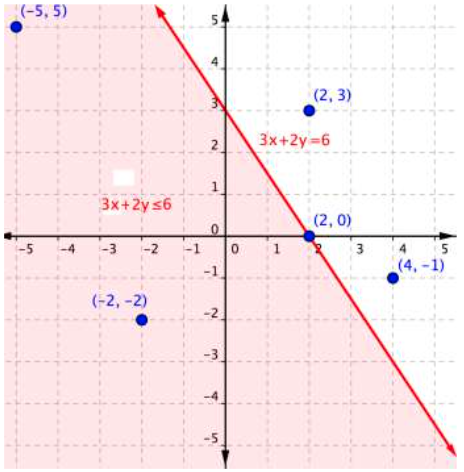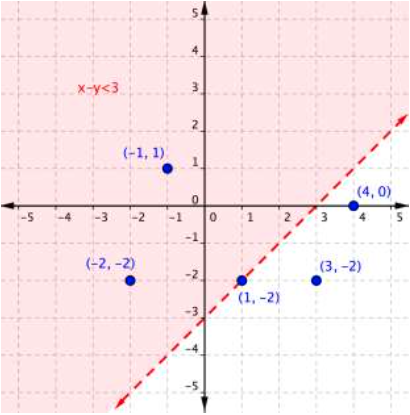## Solution Sets of Inequalities

### Learning Outcome

• Identify whether an ordered pair is in the solution set of a linear inequality

The graph below shows the region of values that makes the inequality $3x+2y\leq6$ true (shaded red), the boundary line $3x+2y=6$, as well as a handful of ordered pairs. The boundary line is solid because points on the boundary line $3x+2y=6$ will make the inequality $3x+2y\leq6$ true.You can substitute the x and y-values of each of the $(x,y)$ ordered pairs into the inequality to find solutions. Sometimes making a table of values makes sense for more complicated inequalities.

Ordered Pair Makes the inequality $3x+2y\leq6$ a true statement Makes the inequality $3x+2y\leq6$ a false statement
$(−5, 5)$ $\begin{array}{r}3\left(−5\right)+2\left(5\right)\leq6\\−15+10\leq6\\−5\leq6\end{array}$
$(−2,−2)$ $\begin{array}{r}3\left(−2\right)+2\left(–2\right)\leq6\\−6+\left(−4\right)\leq6\\–10\leq6\end{array}$
$(2,3)$ $\begin{array}{r}3\left(2\right)+2\left(3\right)\leq6\\6+6\leq6\\12\leq6\end{array}$
$(2,0)$ $\begin{array}{r}3\left(2\right)+2\left(0\right)\leq6\\6+0\leq6\\6\leq6\end{array}$
$(4,−1)$ $\begin{array}{r}3\left(4\right)+2\left(−1\right)\leq6\\12+\left(−2\right)\leq6\\10\leq6\end{array}$

If substituting $(x,y)$ into the inequality yields a true statement, then the ordered pair is a solution to the inequality, and the point will be plotted within the shaded region or the point will be part of a solid boundary line. A false statement means that the ordered pair is not a solution, and the point will graph outside the shaded region, or the point will be part of a dotted boundary line.

### Example

Use the graph to determine which ordered pairs plotted below are solutions of the inequality $x–y<3$.The following video shows an example of determining whether an ordered pair is a solution to an inequality.

### Example

Is $(2,−3)$ a solution of the inequality $y<−3x+1$?

The following video shows another example of determining whether an ordered pair is a solution to an inequality.